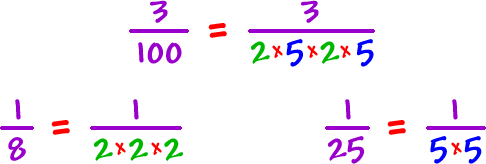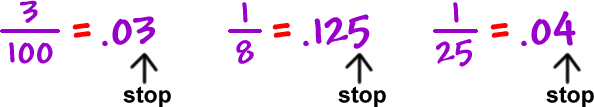In a previous lesson, I showed you how to convert fractions into decimals...  Well, I showed you how to convert SOME fractions into decimals.

The fractions we learned how to change into fractions were special.

Their denominators factored into
ONLY 2's and 5's!

Like these:When you convert these special fractions into decimals, you get terminating decimals!

 Terminating decimals are decimals that stop. They end!Think all decimals do this?

Well, check out the next lesson!